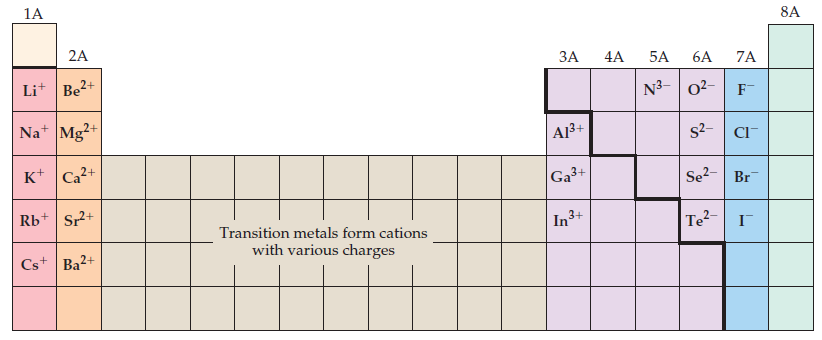Introductory Chemistry - 5 Edition - Chapter 9 - Problem 96p
Register Now

Join StudySoup

Get Full Access to Introductory Chemistry - 5 Edition - Chapter 9 - Problem 96p9780321910295

# Examine Figure 1, which shows the elements that form predictable ions. Use what you know

Introductory Chemistry | 5th Edition

Problem 96P

Examine Figure $$4.14$$, which shows the elements that form predictable ions. Use what you know about electron configurations to explain these trends.

Figure $$4.14$$ Elements that form predictable ionsEquation Transcription:Text Transcription:

4.14

Accepted Solution
Step-by-Step Solution:

Step 1 of 2

The Periodic table, as we all know, is a table in which elements are grouped in series according to their atomic number. The periodic table serves as a basis for determining an element's electrical configuration, physical properties, and chemical properties.

###### Chapter 9, Problem 96P is Solved

Step 2 of 2

Unlock Textbook Solution